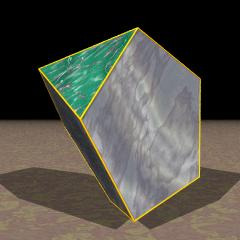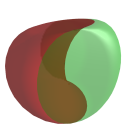Acronym teddi, J63 Name tridiminished icosahedron,ursahedron,vertex figure of sadi `© ©` Circumradius sqrt[(5+sqrt(5))/8] = 0.951057 Inradiuswrt. {3} sqrt[(7+3 sqrt(5))/24] = 0.755761 Inradiuswrt. {5} sqrt[(5+sqrt(5))/40] = 0.425325 Vertex figures [3,52], [33,5] Volume [15+7 sqrt(5)]/24 = 1.277186 Surface [5 sqrt(3)+3 sqrt(25+10 sqrt(5))]/4 = 7.326496 General of army (is itself convex) Colonel of regiment (is itself locally convex) Dihedral angles between {3} and {3}:   arccos(-sqrt(5)/3) = 138.189685° between {3} and {5}:   arccos(-sqrt[(5-2 sqrt(5))/15]) = 100.812317° between {5} and {5}:   arccos(1/sqrt(5)) = 63.434949° Dual titdi Confer uniform relative: ike   related Johnson solids: peppy   gyepip   mibdi   auteddi   faceting of: ike   related CRF: bidex   related orbiform: bitdi   general polytopal classes: Johnson solids   subsymmetrical diminishings   bistratic lace towers   analogs: ursatope Un ExternallinksAs abstract polytope teddi is isomorph to targi, thereby replacing pentagons and pentagrams.

Its name ursahedron is a posterior nickname, derived from its Bowers style acronym teddi. – It furthermore expands to a whole class of related polytopes, the ursatopes.

This polyhedron is an edge-faceting of the icosahedron (ike).

Incidence matrix according to Dynkin symbol

```xfo3oox&#xt   → height(1,2) = sqrt[(3+sqrt(5))/6] = 0.934172
height(2,3) = 1/sqrt(3) = 0.577350
({3} || pseudo f-{3} || dual {3})

o..3o..     | 3 * * | 2 1 0 0 | 1 2 0 0
.o.3.o.     | * 3 * | 0 1 2 0 | 0 2 1 0
..o3..o     | * * 3 | 0 0 2 2 | 0 1 2 1
------------+-------+---------+--------
x.. ...     | 2 0 0 | 3 * * * | 1 1 0 0
oo.3oo.&#x  | 1 1 0 | * 3 * * | 0 2 0 0  wedges
.oo3.oo&#x  | 0 1 1 | * * 6 * | 0 1 1 0
... ..x     | 0 0 2 | * * * 3 | 0 0 1 1
------------+-------+---------+--------
x..3o..     | 3 0 0 | 3 0 0 0 | 1 * * *
xfo ...&#xt | 2 2 1 | 1 2 2 0 | * 3 * *
... .ox&#x  | 0 1 2 | 0 0 2 1 | * * 3 *
..o3..x     | 0 0 3 | 0 0 0 3 | * * * 1
```